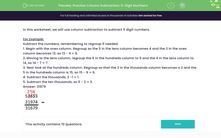# Practise Column Subtraction with 5-Digit Numbers

In this worksheet, students will practise subtracting one five-digit number from another using columns.Key stage:  KS 2

Curriculum topic:   Number: Addition, Subtraction, Multiplication and Division

Curriculum subtopic:   Carry Out Calculations Using Order of Operations

Difficulty level:#### Worksheet Overview

In this worksheet, we will use column subtraction to subtract 5-digit numbers.

For Example:

Subtract the numbers, remembering to exchange if needed:

53,653 - 21,974

1. Begin with the ones column. Exchange so the 5 in the tens column becomes 4 and the 3 in the ones column becomes 13, so 13 - 4 = 9.

2. Moving to the tens column, exchange so that the 6 in the hundreds column becomes 5 and the 4 in the tens column becomes 14, so 14 - 7 = 7.

3. Next look at the hundreds column. Exchange so that the 3 in the thousands column becomes a 2 and the 5 in the hundreds column becomes 15, so 15 - 9 = 6.

4. Subtract the thousands 2 - 1 = 1.

5. Subtract the ten thousands 5 - 2 = 3.The example above involved quite a lot  of exchanging between the columns, so you can use this to refer back to if you find any of the following questions tricky.

### What is EdPlace?

We're your National Curriculum aligned online education content provider helping each child succeed in English, maths and science from year 1 to GCSE. With an EdPlace account you’ll be able to track and measure progress, helping each child achieve their best. We build confidence and attainment by personalising each child’s learning at a level that suits them.

Get started